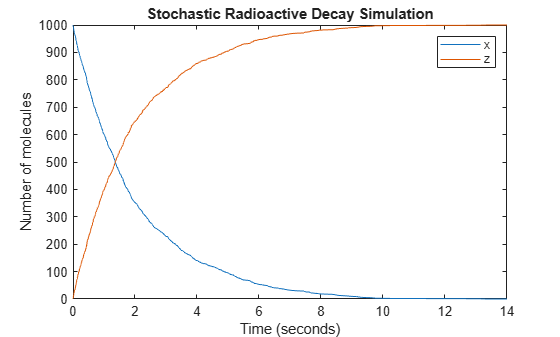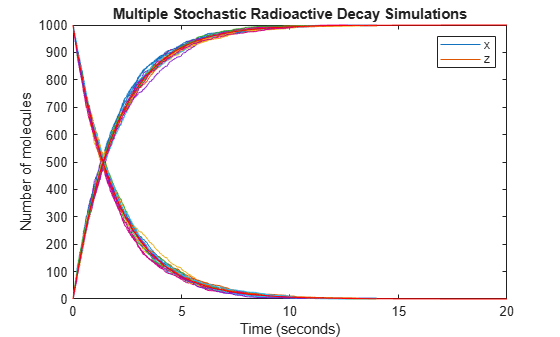# Stochastic Simulation of Radioactive Decay

This example shows how to build and simulate a model using the SSA stochastic solver.

The following model will be constructed and stochastically simulated:

• Reaction 1: x -> z with a first-order reaction rate, c = 0.5.

• Initial conditions: x = 1000 molecules, z = 0.

This model can also be used to represent irreversible isomerization.

This example uses parameters and conditions as described in Daniel T. Gillespie, 1977, "Exact Stochastic Simulation of Coupled Chemical Reactions," The Journal of Physical Chemistry, vol. 81, no. 25, pp. 2340-2361.

SBML = Systems Biology Markup Language, www.sbml.org

`model = sbmlimport('radiodecay.xml')`
```model = SimBiology Model - RadioactiveDecay Model Components: Compartments: 1 Events: 0 Parameters: 1 Reactions: 1 Rules: 0 Species: 2 Observables: 0 ```

### View Species Objects of the Model

`model.Species`
```ans = SimBiology Species Array Index: Compartment: Name: Value: Units: 1 unnamed x 1000 molecule 2 unnamed z 0 molecule ```

### View Reaction Objects of the Model

`model.Reactions`
```ans = SimBiology Reaction Array Index: Reaction: 1 x -> z ```

### View Parameter Objects for the Kinetic Law

`model.Reactions(1).KineticLaw(1).Parameters`
```ans = SimBiology Parameter Array Index: Name: Value: Units: 1 c 0.5 1/second ```

### Update the Reaction to use MassAction Kinetic Law for Stochastic Solvers.

```model.Reactions(1).KineticLaw(1).KineticLawName = 'MassAction'; model.Reactions(1).KineticLaw(1).ParameterVariableNames = {'c'};```

### Simulate the Model Using the Stochastic (SSA) Solver & Plot

```cs = getconfigset(model,'active'); cs.SolverType = 'ssa'; cs.StopTime = 14.0; cs.CompileOptions.DimensionalAnalysis = false; [t,X] = sbiosimulate(model); plot(t,X); legend('x', 'z', 'AutoUpdate', 'off'); title('Stochastic Radioactive Decay Simulation'); ylabel('Number of molecules'); xlabel('Time (seconds)');```### Repeat the Simulation to Show Run-to-Run Variability

```title('Multiple Stochastic Radioactive Decay Simulations'); hold on; for loop = 1:20 [t,X] = sbiosimulate(model); plot(t,X); % Just plot number of reactant molecules drawnow; end```### Overlay the Reaction's ODE Solution in Red

```cs = getconfigset(model,'active'); cs.SolverType = 'sundials'; cs.StopTime = 20; [t,X] = sbiosimulate(model); plot(t,X,'red'); hold off;```